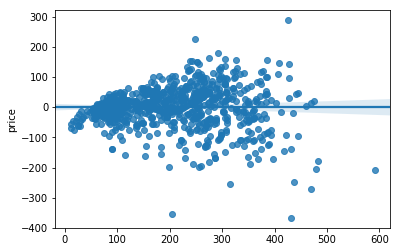Linear Regression

### Math behind the Algorithm

Linear Regression is a common algorithm used to predict a continuous value (like housing prices) using a number of features (neighborhood, bedrooms, etc...) by fitting a line that most accurately represents the data. This is achieved by taking the sum of each feature multiplied by a weight and a separate bias (constant value) value added. The equation looks as followed:

$$\hat{y}=\theta_0 + \theta_1x_1 + \theta_2x_2 + ... + \theta_nx_n$$

As you can see there is the bias value denoted as $\theta_0$ followed by each feature value ($x_1$ to n) multiplied by a weight ($\theta_1$to n).

This can be written more concisely using vectorized form shown below. This is just the dot product of the transpose (row instead of column vector) of the $\theta$ values and the x values in vector format:

$$\hat{y}=h_\theta(\textbf{x}) = \theta^T \cdot \textbf{x}$$### Cost Function

The next step is to find the weights for each feature that will create the best fit on the dataset. This is done with the help of a cost function. With linear regression mean squared error (MSE) is usually used and the goal is find the best $\theta$ value that reduces the MSE. As its name implies MSE determines the error by first taking the difference between the guessed value and actual value, squaring this value, and then taking the average of all the values. The MSE equation is shown below:

$$MSE(\textbf{X}, h_\theta) = \frac{1}{m} \sum_{i=1}^m (\theta^T \cdot \textbf{x}^{(i)} - y^{(i)})^2$$### Minimizing the Cost Function

Now to actually find these weights there are two different approaches. The first is called the Normal Equation which is a closed-form solution. This means that all you need to do is plug the values into the equation to get the correct weights.

Normal Equation:

$$\hat{\theta}=(\textbf{X}^T \cdot \textbf{X})^{-1} \cdot \textbf{X}^T \cdot \textbf{y}$$

The other approach is by using an optimization algorithm called gradient descent. Unlike the normal equation this is an iterative approach. The reason gradient descent may be used is because if there are a large number of features or too many instances to fit into memory then the normal equation is going to take to long to find the $\theta$ values.

To get a deeper look into gradient descent the Gradient Descent section has an in depth look at variations of it and some of the math behind it.

### Scikit-Learn - Linear Regression

Next will be a look at an implementation of Linear Regression using Scikit-Learn.

In :
from sklearn.linear_model import LinearRegression
from sklearn.pipeline import Pipeline
from sklearn.preprocessing import OneHotEncoder
from sklearn.preprocessing import StandardScaler

from sklearn.model_selection import train_test_split
from sklearn.model_selection import cross_val_score

import numpy as np
import pandas as pd

# grabbing the data and dropping the columns that will not be used or have missing data
data = data.drop(['borough', 'last_modified', 'room_id','host_id'], axis=1)
data = data.dropna()

# separate features and target columns
X = data.drop('price', axis=1)
y = data['price']

# split the data into a training and test dataset
X_train, X_test, y_train, y_test = train_test_split(X, y, test_size=0.2, random_state=50)

In :
train_data = X_train.copy()

# Here are categorical columns that need to be hot encoded
# pd.get_dummies(data_cat) is another way of achieving this and is
# a good way to see how one hot encoding works
data_cat = train_data[['room_type', 'neighborhood']]

# OneHotEncoder does not take strings so the string categories need to be
# changed to integer values with the factorize() method
data_cat_to_int = data_cat.apply(lambda x: x.factorize())

# Each variable has two columns that need to be hot encoded.  Reshape is neccessary
# because the encoder wants a column vector of the values.
encoder = OneHotEncoder()
data_cat_encoded = data_cat_to_int.apply(lambda x: encoder.fit_transform(x.reshape(-1,1)))

In :
# drop the categorical columns
data_num = train_data.drop(['room_type','neighborhood'], axis=1)

# standardize the data, more important when using gradient descent or
# regularization methods
std = StandardScaler()
data_num = std.fit_transform(data_num)

training_data = np.concatenate([data_num, data_cat_encoded.toarray(),
data_cat_encoded.toarray()], axis=1)

In :
# fit onto the training data
linear_regression = LinearRegression()
linear_regression.fit(training_data, y_train)

# linear_regression.intercept_, linear_regression.coef_

Out:
LinearRegression(copy_X=True, fit_intercept=True, n_jobs=1, normalize=False)
In :
# k folds is a good way to see the error on the data
scores = cross_val_score(linear_regression, training_data, y_train,
scoring="neg_mean_squared_error", cv=10)
lin_reg_rsme_scores = np.sqrt(-scores)
print(lin_reg_rsme_scores)

[ 70.03524077  71.64582723  83.49986908  67.46343742  79.31534962
73.13416946  68.20696759  60.37043868  56.98559473  86.28481587]

In :
# see how the training data predictions compare to the actual predictions
y_train_pred = linear_regression.predict(training_data)

from sklearn.metrics import mean_squared_error, r2_score
mean_squared_error(y_train, y_train_pred)
r2_score(y_train, y_train_pred)

Out:
0.70114458356406562
In :
import seaborn as sns
%matplotlib inline

sns.regplot(x=y_train_pred, y=(y_train_pred - y_train))

Out:
<matplotlib.axes._subplots.AxesSubplot at 0x7f93bfa5f0b8>Resources:

1. Hands-On Machine Learning with Scikit-Learn & Tensorflow by Aurelien Geron
2. Python Machine Learning by Sebastian Raschka and Vahid Mirjalili
3. Scikit-Learn Documentation